### 东风悦达起亚 起亚KX5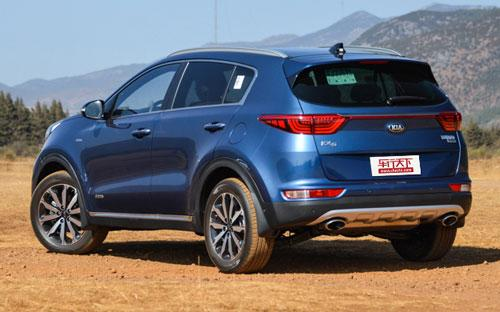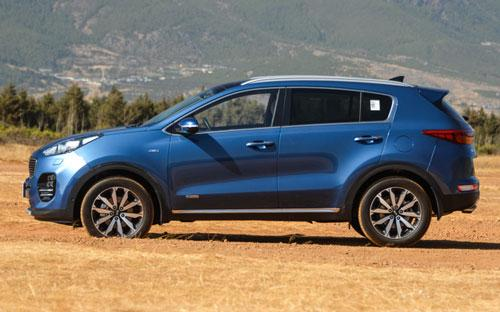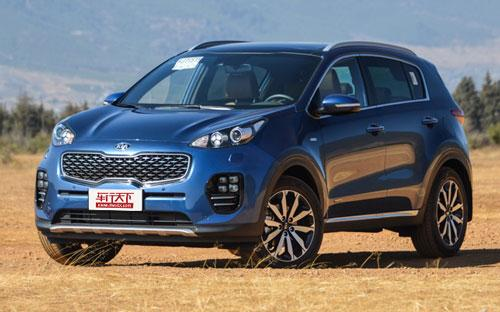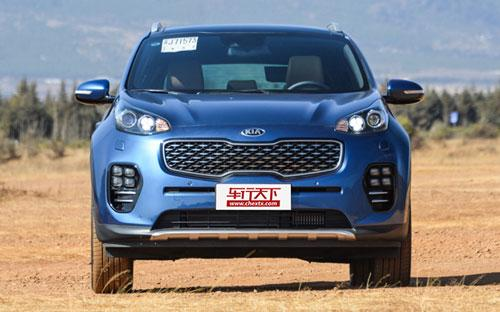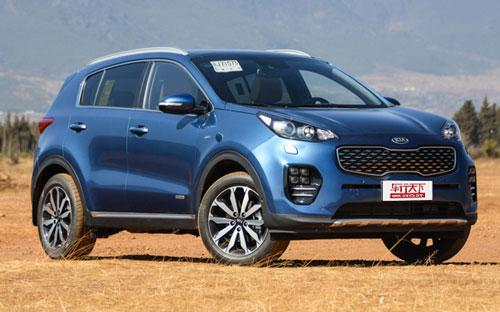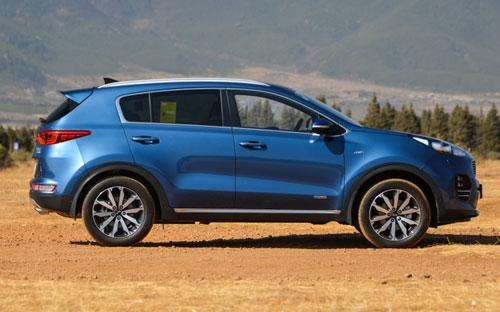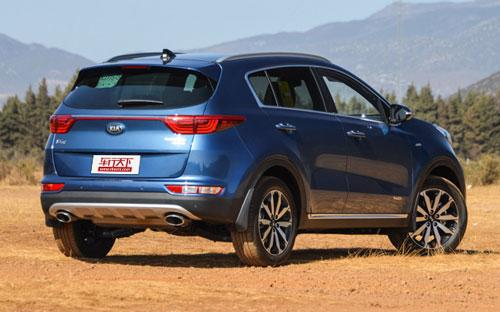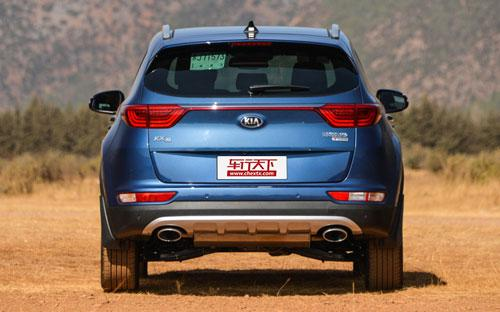7 种颜色可选2016款最低售价：15.68 万元起

4480(mm)1855(mm)1655(mm)##### 配置亮点：
• 胎压监测装置

• ISOFIX儿童座椅接口

• 车身稳定控制(ESC/ESP/DSC等)

• 电动天窗

• 定速巡航

• 后倒车雷达

• 真皮座椅

• GPS导航系统

• 氙气大灯

• 后视镜加热

• 提交
• 东风悦达起亚 起亚KX5 绕车实拍• 东风悦达起亚 起亚KX5 在售车型

排量 车型 厂商指导价 本地最低报价 购车工具
1.6L
2.0L 手动两驱GL 6挡手动
15.68万
15.68万

2.0L 自动两驱GL 6挡自动
16.18万
17.18万
17.18万

18.98万
1.6T 自动两驱GL 7挡双离合
16.68万
1.6T 自动两驱DLX 7挡双离合
17.68万
2.0L
2.0L 手动两驱GL 6挡手动
15.68万
15.68万

2.0L 自动两驱GL 6挡自动
16.18万
17.18万
17.18万

18.98万
1.6T 自动两驱GL 7挡双离合
16.68万
1.6T 自动两驱DLX 7挡双离合
17.68万
1.6T
19.38万
23.18万
23.18万

东风悦达起亚 起亚KX5 经销商

查看更多 >>

### 东风悦达起亚 起亚KX5 动力加速

起亚KX5 0-100公里加速时间分布在 0.0-秒 属于 超跑级

动力级别 加速时间 车型

东风悦达起亚 起亚KX5 视频

东风悦达起亚 起亚KX5 新闻资讯

# 猴年就要猴赛雷 河南万盛起亚KX5到店实拍

新闻 超过8409次关注

东风悦达起亚KX5在去年广州车展首次亮相，它与现代途胜属同平台打造。定位为紧凑型SUV根据之前的报道，KX5将会于3月10日正式上市销售。

# 起亚KX5配置高规格 挑战日系

国产新车 超过8452次关注

起亚KX5预计于3月10日上市，新车的动力和配置相比老款起亚智跑有了一定提升，采用1.6T发动机取代智跑的2.4L发动机，匹配7速双离合变速箱；新增发动机自动启停、自适...

# 猴年春节过后国内首批上市新车一览

即将上市 超过9419次关注

猴年开门红！春节后就有不少新车迫不及待的要上市啦。其中包括非常热门的迈锐宝XL、国产起亚KX5等等。

# 两种排量八款车型 起亚KX5详细配置曝光

即将上市 超过8518次关注

近日，我们从相关渠道，起亚旗下SUV东风悦达KX5有望于3月10日正式上市，国产起亚KX5将提供2.0L和1.6T两款发动机，匹配6挡手动、6挡自动、7挡双离合三种变速箱，共计八款车型。

猜你喜欢

﻿
• 快速找车
• 选择品牌
• 选择品牌
• A  奥迪
• A  阿斯顿·马丁
• A  阿尔法·罗密欧
• B  宝沃
• B  布加迪
• B  巴博斯
• B  保时捷
• B  宾利
• B  奔驰
• B  宝马
• B  本田
• B  别克
• B  标致
• B  比亚迪
• B  宝骏
• B  北汽制造
• B  北汽新能源
• B  北汽幻速
• B  北汽威旺
• B  北京汽车
• B  奔腾
• B  北汽绅宝
• C  长安
• C  长安商用
• C  长城
• C  昌河
• D  大众
• D  道奇
• D  DS
• D  东南
• D  东风风神
• D  东风风行
• D  东风小康
• D  东风风度
• D  东风
• F  福特
• F  丰田
• F  菲亚特
• F  法拉利
• F  福田
• F  福迪
• F  福汽启腾
• G  观致
• G  广汽传祺
• G  广汽吉奥
• G  GMC
• H  红旗
• H  汉腾汽车
• H  哈弗
• H  哈飞
• H  海格
• H  海马
• H  华颂
• H  黄海
• H  华泰
• H  恒天
• J  吉利汽车
• J  捷豹
• J  Jeep
• J  江淮
• J  江铃
• J  金杯
• J  九龙
• J  金旅
• K  凯翼
• K  凯迪拉克
• K  克莱斯勒
• K  科尼塞克
• K  卡威
• K  开瑞
• L  路虎
• L  林肯
• L  劳斯莱斯
• L  兰博基尼
• L  雷克萨斯
• L  铃木
• L  雷诺
• L  理念
• L  力帆
• L  莲花汽车
• L  猎豹
• L  路特斯
• L  陆风
• M  马自达
• M  MG
• M  MINI
• M  玛莎拉蒂
• M  摩根
• M  迈凯轮
• N  纳智捷
• O  欧宝
• O  讴歌
• O  欧朗
• Q  奇瑞
• Q  起亚
• Q  启辰
• R  日产
• R  荣威
• R  瑞麒
• S  三菱
• S  斯威汽车
• S  萨博
• S  smart
• S  斯柯达
• S  斯巴鲁
• S  思铭
• S  双龙
• S  上汽大通
• S  双环
• T  特斯拉
• T  腾势
• W  沃尔沃
• W  五菱汽车
• W  五十铃
• W  威兹曼
• W  威麟
• X  现代
• X  雪佛兰
• X  雪铁龙
• X  西雅特
• Y  一汽
• Y  英菲尼迪
• Y  英致
• Y  依维柯
• Y  野马汽车
• Y  永源
• Z  众泰
• Z  中华
• Z  中兴
• Z  知豆
• 选择车系
• 选择车系
• 车型对比
• 选择品牌
• 选择品牌
• A  奥迪
• A  阿斯顿·马丁
• A  阿尔法·罗密欧
• B  宝沃
• B  布加迪
• B  巴博斯
• B  保时捷
• B  宾利
• B  奔驰
• B  宝马
• B  本田
• B  别克
• B  标致
• B  比亚迪
• B  宝骏
• B  北汽制造
• B  北汽新能源
• B  北汽幻速
• B  北汽威旺
• B  北京汽车
• B  奔腾
• B  北汽绅宝
• C  长安
• C  长安商用
• C  长城
• C  昌河
• D  大众
• D  道奇
• D  DS
• D  东南
• D  东风风神
• D  东风风行
• D  东风小康
• D  东风风度
• D  东风
• F  福特
• F  丰田
• F  菲亚特
• F  法拉利
• F  福田
• F  福迪
• F  福汽启腾
• G  观致
• G  广汽传祺
• G  广汽吉奥
• G  GMC
• H  红旗
• H  汉腾汽车
• H  哈弗
• H  哈飞
• H  海格
• H  海马
• H  华颂
• H  黄海
• H  华泰
• H  恒天
• J  吉利汽车
• J  捷豹
• J  Jeep
• J  江淮
• J  江铃
• J  金杯
• J  九龙
• J  金旅
• K  凯翼
• K  凯迪拉克
• K  克莱斯勒
• K  科尼塞克
• K  卡威
• K  开瑞
• L  路虎
• L  林肯
• L  劳斯莱斯
• L  兰博基尼
• L  雷克萨斯
• L  铃木
• L  雷诺
• L  理念
• L  力帆
• L  莲花汽车
• L  猎豹
• L  路特斯
• L  陆风
• M  马自达
• M  MG
• M  MINI
• M  玛莎拉蒂
• M  摩根
• M  迈凯轮
• N  纳智捷
• O  欧宝
• O  讴歌
• O  欧朗
• Q  奇瑞
• Q  起亚
• Q  启辰
• R  日产
• R  荣威
• R  瑞麒
• S  三菱
• S  斯威汽车
• S  萨博
• S  smart
• S  斯柯达
• S  斯巴鲁
• S  思铭
• S  双龙
• S  上汽大通
• S  双环
• T  特斯拉
• T  腾势
• W  沃尔沃
• W  五菱汽车
• W  五十铃
• W  威兹曼
• W  威麟
• X  现代
• X  雪佛兰
• X  雪铁龙
• X  西雅特
• Y  一汽
• Y  英菲尼迪
• Y  英致
• Y  依维柯
• Y  野马汽车
• Y  永源
• Z  众泰
• Z  中华
• Z  中兴
• Z  知豆
• 选择车系
• 选择车系
• 选择车型
• 选择车型
• 意见反馈